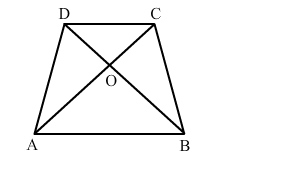# In the given figure, ABCD is a trapezium whose diagonals AC and BD intersect at O such that OA = (3x −1)Question:

In the given figure, ABCD is a trapezium whose diagonals AC and BD intersect at O such that OA = (3x −1) cm, OB = (2x + 1) cm, OC = (5x − 3) cm and OD = (6x − 5) cm. Then, x = ?(a) 2
(b) 3
(c) 2.5
(d) 4

Solution:

(a) 2

We know that the diagonals of a trapezium are proportional.
Therefore,

$\frac{O A}{O C}=\frac{O B}{O D}$

$\Rightarrow \frac{3 X-1}{5 X-3}=\frac{2 X+1}{6 X-5}$

$\Rightarrow(3 X-1)(6 X-5)=(2 X+1)(5 X-3)$

$\Rightarrow 18 X^{2}-15 X-6 X+5=10 X^{2}-6 X+5 X-3$

$\Rightarrow 18 X^{2}-21 X+5=10 X^{2}-X-3$

$\Rightarrow 18 X^{2}-21 X+5-10 X^{2}+X+3=0$

$\Rightarrow 8 X^{2}-20 X+8=0$

$\Rightarrow 4\left(2 X^{2}-5 X+2\right)=0$

$\Rightarrow 2 X^{2}-5 X+2=0$

$\Rightarrow 2 X^{2}-4 X-X+2=0$

$\Rightarrow 2 X(X-2)-1(X-2)=0$

$\Rightarrow(X-2)(2 X-1)=0$

$\Rightarrow$ Either $x-2=0$ or $2 x-1=0$

$\Rightarrow$ Either $x=2$ or $x=\frac{1}{2}$

When $x=\frac{1}{2}, 6 x-5=-2<0$, which is not possible.

Therefore, $x=2$